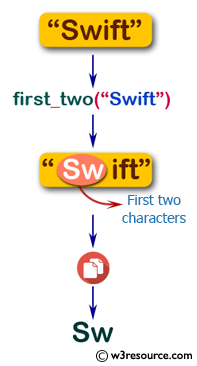﻿ Swift String Programming Exercise: Create a new string made of a copy of the first two characters of a given string - w3resource# Swift String Exercises: Create a new string made of a copy of the first two characters of a given string

## Swift String Programming: Exercise-4 with Solution

Write a Swift program to create a new string made of a copy of the first two characters of a given string. If the given string is shorter than length 2, return whatever there is.

Pictorial Presentation:Sample Solution:

Swift Code:

``````import Foundation
func first_two(_ input: String) -> String {
let chars = input.characters
let firstTwo = chars.prefix(2)

return String(firstTwo)
}
print(first_two("Swift"))
print(first_two("Sw"))
print(first_two(""))
print(first_two("S"))
```
```

Sample Output:

```
Sw
Sw

S
```

Swift Programming Code Editor:

Improve this sample solution and post your code through Disqus

What is the difficulty level of this exercise?

﻿